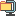검색어 입력폼

Multivariate Statistical Analysis LDA,QDA, decision tree

저작시기 2012.04 |등록일 2012.05.08압축파일 (zip) | 13페이지 | 가격 1,000원

소개글

주어진 데이터를 가지고 R프로그램에서
LDA, QDA, decision tree 만들고,
이의 sensitivity, specificity, 10-fold cross validation, information gain,
sum of squared error 을 계산한 레포트 입니다.

목차

1. Consider the following two-class data.
2. Consider the dataset (Cleveland Heart Disease Dataset.xls) and its description (Cleveland Heart Disease.doc) that I upload in the portal system. Split the dataset into two parts as follows:
3. Consider the dataset (ToyotaCorolla.xls) that I upload in the portal system.

본문내용

1. Consider the following two-class data.

library(MASS) #이것을 해야 linear discriminant boundary와 quadratic discriminant boundary

(1) Find the linear discriminant boundary based on the normal distribution and draw a sketch of the decision boundary.
m<- lda(Y~ ., data = data, prior = c(1, 1)/2, CV=TRUE) # = m<-lda(Y~ ., data = data)
ct<-table(data\$Y, m\$class)
sum(diag(prop.table(ct)))

plot(m, dimen=1, type="both") #(1)

library(klaR)
levels(data\$Y)<- c(`1`, `2`)
partimat(Y~X1+X2,data=data,method="lda") #(2)

pairs(data[c("X1","X2")], main="My Title ", pch=22,
bg=c("red", "yellow")[unclass(data\$Y)]) #(3)

없음

압축파일 내 파일목록

Cleveland Heart Disease Dataset.xlsx
Cleveland Heart Disease Description.doc
Multivariate Statistical Analysis.hwp
ToyotaCorolla.csv